# Design of feed systems for hologram-based compact antenna test ranges

N/A
N/A
Info
Protected

Jaa "Design of feed systems for hologram-based compact antenna test ranges "

Copied!
106
0
0

Kokoteksti

Name of the thesis: Design of feed systems for hologram-based compact antenna test arrays. In this thesis, the design principle of the feeding systems for hologram-based compact series is presented.

## Far-field measurement

In section 8.1 the 650 GHz feed horns are measured in the far field of the AUT. The 650 GHz feed horns, in section 8.1, are measured in the far field of the AUT.

## Near-field measurement

The far field criterion is defined as the distance from the antenna where is the distance to the edge of the antenna. The far-field model was not calculated since DRFS is used in the near-field region (the far-field criterion gives a few hundred meters and the distance to the hologram is 12.72 m).

## Compact antenna test range

### Reflector-based compact antenna test range

Planarity errors can be corrected based on the measurement results if the scanner's planarity is known. The cross-polarization performance can be improved by using two reflectors and by choosing the parameters of the CATR so that the cross-polarization is minimized , .

### Lens-based compact antenna test range

The range of compact antennas based on a lens is mainly possible at very high frequencies as the surface accuracy requirement for a reflector becomes very strict. Because a lens is a transmission-type element and because the wavelength is shorter inside the lens, the surface accuracy requirement is weighted by  r 1 2 compared to a reflector .

### Hologram-based compact antenna test range

• History of antenna tests in a hologram-based CATR
• Feed system for a hologram-based CATR

A DRFS is used to provide customized illumination for the hologram for the first time during an antenna measurement. The remote supply, i.e. the DRFS, is placed 12.72 m from the hologram and the AUT is placed approximately 9 m from the hologram.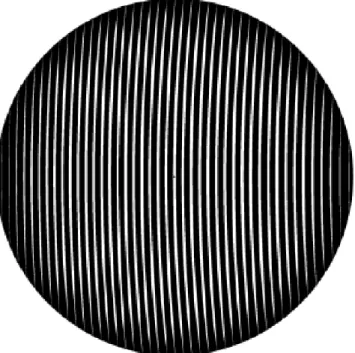Figure 2.6: Example of a transmission-type amplitude hologram pattern. Metal is in black and slots in white

The antenna structure can be replaced with equivalent current sources without changing the radiated field. When the equivalent current sources are determined, the radiated field can be calculated with Maxwell's equations.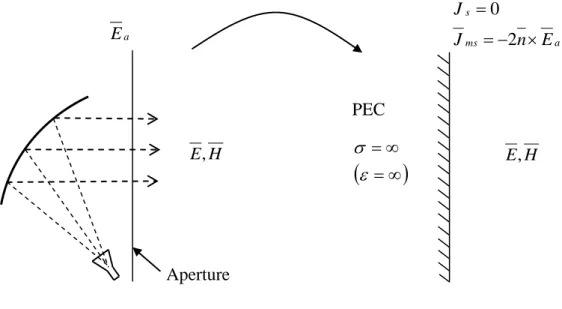Figure 3.1: The original antenna problem is replaced with perfect conductor and equivalent magnetic surface currents

## Physical optics

In the shadow region, i.e. surface area not directly illuminated by the incident field, the surface currents are assumed to be zero. At these points the surface is approximated with the tangential plane and surface currents are calculated from (3.19).

## Physical theory of diffraction

The number of current elements must be large enough for the PO to provide accurate prediction of the reflected field. The required number of current elements depends on the size and shape of the object compared to the wavelength and the desired field accuracy.

## Geometrical optics

If E0 is known at some point, it can be solved for at all points along the ray. Power spreads within the jet tube and the power density depends on the cross-sectional area of ​​the jet tube.

## Reflector antennas

### Rotated conic sections

Large reflector and lens antennas are aperture antennas used to redirect the radiation from a primary feed. Reflector and lens antennas can be divided into common antenna types and shaped antennas.

### Collimating reflector antennas

The paraboloid can be fed directly from the focal point or a subreflector antenna can be used whose focal point coincides with the focal point of the paraboloid. The cross-polarization caused by the offset structure can be minimized with the so-called compensated design based on the Mizugutch condition .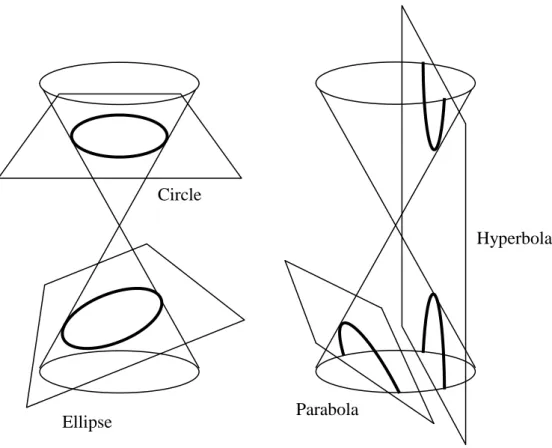Figure 4.1: A conic section is an intersection of a plane and one or two napes of a cone

### Diverging-beam reflector antennas

The dual reflector feed systems, in Chapter 6, are based on the dual offset hyperboloid-hyperboloid geometry. For both sub and main reflectors, one focal point is behind the reflector surface, therefore the geometry is similar to the hyperboloid-hyperboloid geometry.

## Lens antennas

The eccentricity should be equal to 1/n, where n is the refractive index of the lens material . Examples of the synthesized ellipsoid with an elongated hemispherical lens and a true ellipsoid lens are illustrated in Figure 4.7. An ideal Luneburg lens is a sphere with a different relative permittivity that follows the following equation: .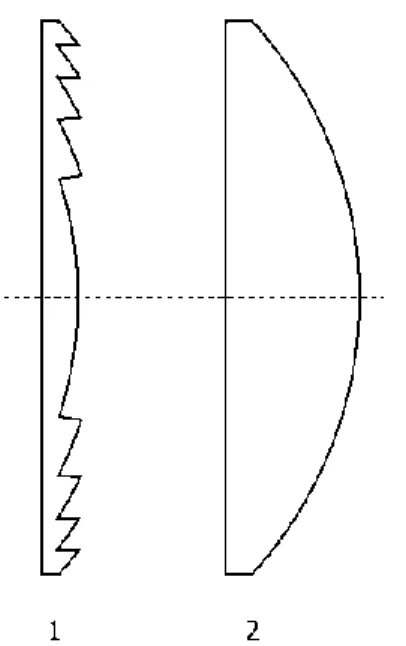Figure 4.6: Examples of dielectric lens antennas; 1) Fresnel, and 2) hyperboloid.

Shaped antennas

## Synthesis methods for shaped antennas

• Reflector synthesis methods
• Ray-tracing based reflector synthesis methods
• Substrate lens synthesis methods
• Dielectric lens synthesis methods

The method is used to design a double reflector feed system (DRFS) for a single reflector CATR. In  this method is used for a 3D shaped lens interpolated from two profiles calculated independently for two planes of the lens. GO and two-dimensional integration of the aperture distribution are used to calculate radiation patterns.

## Feed systems for hologram-based CATR

In general, the hologram is not in the far field of the inlet system, so the far field pattern of the inlet system is not of interest. Therefore, the illumination amplitude pattern, that is, the width of the flat-amplitude region, determines the magnitude of QZ. Furthermore, the optimization procedure, as explained in Section 5.2, is an important part of the developed synthesis method.

## Properties of rays and ray-tracing

• Ray, ray tube, and field
• Ray direction
• First-order wave front approximation
• Amplitude, phase, and polarisation along a ray
• Ray direction and known focal point
• Ray direction and field phase
• Power, amplitude and ray tubes
• Reflection and refraction from a planar surface
• Polarisation of reflected and refracted rays

Some beam properties described in this section are not used in the synthesis of control systems. The ray represents the direction of propagation, which is the same as the direction of the local wave vector k(r). The phase derivatives determine the tangential vectors of the plane, i.e. constant phase wavefront of a plane wave.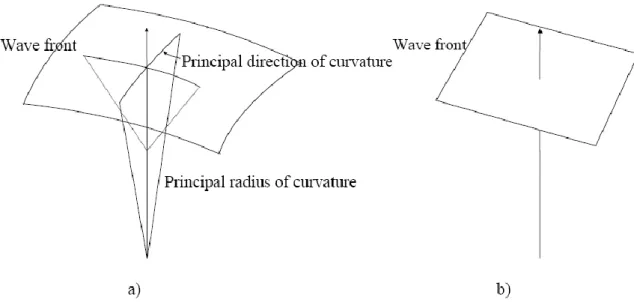Figure 5.1: Local approximation of a wave front; a) second-order and b) first-order approximation 

## Feed system design procedure

In general, first 1) the basic geometry parameters are optimized, then 2) the input and output fields and their ray rendering are optimized, and finally 3) the synthesis parameters are optimized. In practice, the design process is not so straightforward, because all steps in the design process influence each other. In this way it is possible to study separately how each parameter affects the radiation of the antenna.

## Synthesis of a feed system

### Basic geometry

Two focal points: the central point of the feed horn phase and the central point of the supply system. The focal point of the feed system is usually the same as the focal point of the Fholo hologram. Ray center path from the center point of the feed horn phase to the center of the output field aperture, i.e., the hologram.

### Representation of fields with rays

• Input field
• Output field
• Aperture mapping
• Rotationally symmetric aperture mapping

The input beams are mapped to the output beams, that is, the output beam grid is changed so that the power in each beam is the same in the output as in the corresponding input beam. The output field amplitude is defined as a rotationally symmetric function Eout,norm(nray) and the input field is averaged. The output field Eout(nray) is known in a constant ∆' grid (or another user-defined grid).

### Synthesis of the surfaces

The 2nd surface point (nray, mray) is calculated in the same way as the intercept point of the exit ray (nray, mray) and the tangent plane at the leading point i.e. point (nray–1, mray) of the 2nd surface point. surface. The second surface point is then moved along the exit beam to adjust the total length of the beam l(nray, mray) from the phase center of the feed horn to the exit field opening. In the case of a shaped lens delivery system, the desired beam length is an electrical beam length, which takes into account the electrical properties of the lens.

## Simulations

Simulations with GRASP8W

### Ray-tracing simulation

• Ray definition and ray tracing to the aperture
• Calculation of the aperture field
• Calculation of hologram illumination with Huygens’ principle

The vertical polarization, i.e. the main polarization, of the radiation pattern of the feed horn is used in the calculations of the radiator power. Using the expression for the pipe effect in (5.8) is the pipe force at the opening. The input field is defined by the aperture of the input field from the feed horn's far field pattern.

## A 310 GHz DRFS

The focal length of the subreflector fsub is the distance between the feed horn phase center and the center of the subreflector. The focal point of the hologram is Fholo and it is also the focal point of the feeding system. The focal point of the system is pholo and the distance from main reflector to the hologram center is preserved.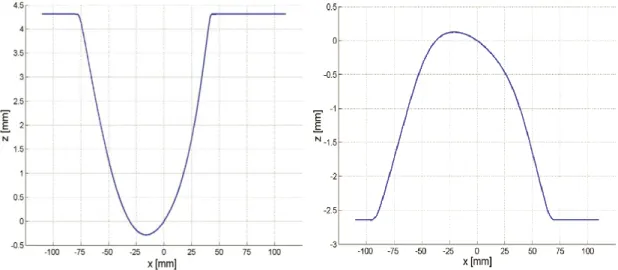Figure 6.2: Horizontal cross-cuts of the subreflector (left-hand-side) and of the main reflector (right-hand-side) 

## Design of a 650 GHz DRFS

• Basic geometry
• Input and output fields and rays
• Synthesised reflector surfaces and mechanical design
• Simulation results
• Comparison of the 650 GHz DRFS to the 310 GHz DRFS

The ratio between the input and output field beamwidths (and shapes) must also be correct. This synthesis error is greater with a wider beam of the synthesis output field. In the 650 GHz DRFS, the edge illumination of the reflectors is lower than in the 310 GHz DRFS.

## Elliptical and hyperbolical DRFS geometries

In the design of the 310 GHz DRFS , the desired hologram illumination and reflector synthesis output field were the same. A shaped lens feed system is used to modify the radiation of the primary feed ie. amplitudes and phases of the corrugated supply horn. The distance between the shaped faces must be relatively large, since the distance between the beams, i.e. the power density, varies between the shaped faces.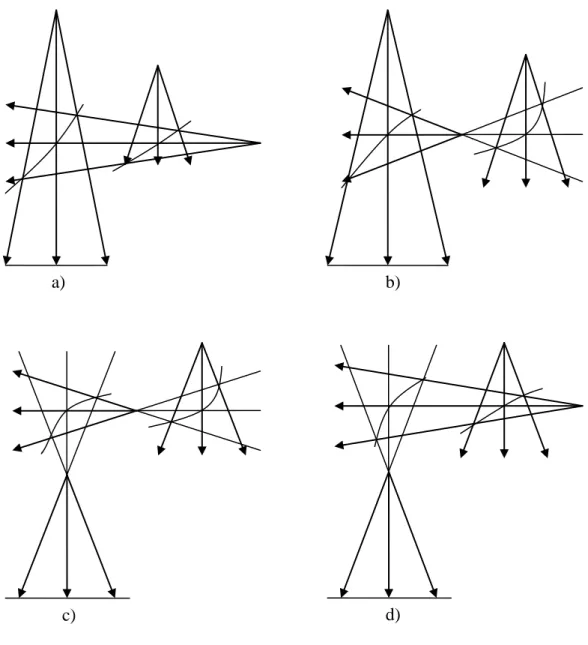Figure 6.15: Examples of possible DRFS basic geometries: a) hyperboloid- hyperboloid-hyperboloid, b) ellipsoid-hyperboloid-hyperboloid, c) ellipsoid-ellipsoid, and d) hyperboloid-ellipsoid

## Dual lens feed system

• Geometry
• Input and output fields in synthesis
• Synthesis and the synthesised surfaces
• Simulated hologram illumination
• Comparison to the 650 GHz DRFS

The geometry of the dual lens feeding system is described in Section 7.1.1, and the input and output fields and beams used in the synthesis are described in 7.1.2. The desired beam length is calculated from (5.36), where the constant C can be calculated from the center beam geometry:. 7.3) The synthesized surfaces and some of the rays are shown in Figure 7.5. All the simulation results in Table 7.2 are in favor of the dual lens advance system.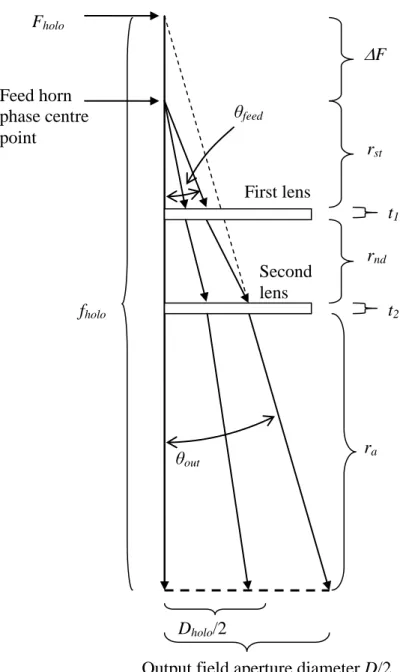Figure 7.2: Basic geometry of a dual lens feed system. The figure is not in scale.

## The 650 GHz feed horns

The 650 DRFS, presented in section 6.2, was used to provide the custom lighting for the hologram. The beam widths of the horns were measured and the results are shown in section 8.1. As shown in Figure 8.1, the beam width of the DRFS feed horn is narrower than the simulated beam width used in the design of the DRFS and in the simulations presented in Section 6.2.

## The 650 GHz DRFS

• Measurement setup
• Error compensation techniques
• Planarity error correction technique
• Measurement results of the 650 GHz DRFS

The measurement uncertainty in the laser tracker interferometer measurements was ±20 μm (with 2 sigma specifications), that is, the planarity correction accuracy would be ±16° at 650 GHz. The parameter optimization is based on the minimization of the average standard deviation of the planarity error-compensated phase deviation patterns in the central region [ 85 ]. The beam shape, i.e. the −1 dB beam width and the hologram edge illumination, are about the same in the measured and the simulated amplitude patterns.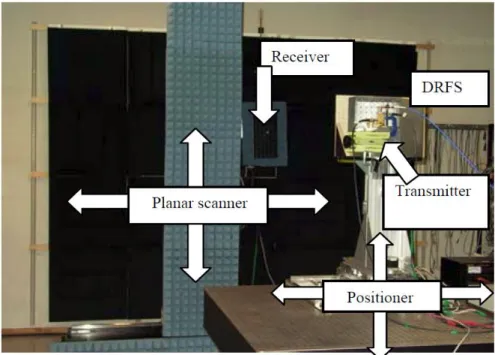Figure 8.2: The measurement setup; the AUT positioner and the planar scanner.

## Hologram-based CATR at 650 GHz

Räisänen, "A compact antenna key sequence based on a hologram," IEEE Transactions on Antennas and Propagation, vol. Räisänen, "Dual reflector feed system for hologram-based compact antenna test range," IEEE Transactions on Antennas and Propagation, vol. Räisänen, "Hologram-based compact array vir submillimeter-wave antenna testing," IEEE Transactions on Antennas and Propagation, vol.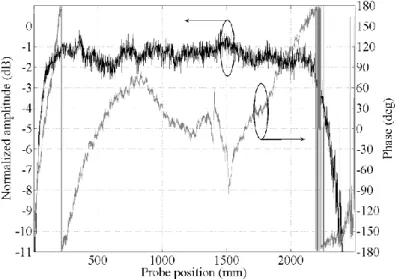Figure 8.7: Horizontal scan of the quiet-zone field. AUT centre at 1290 mm .

Kuvio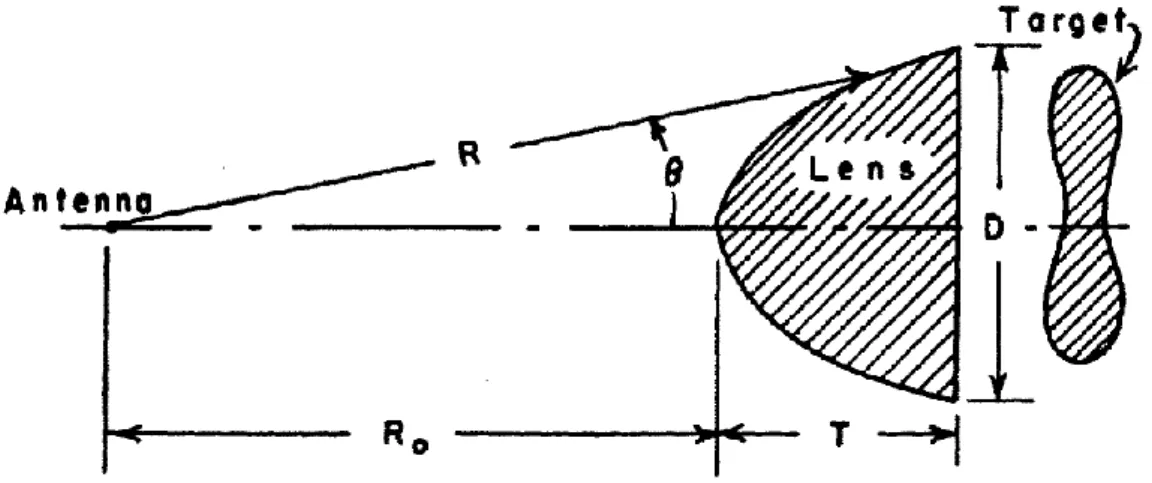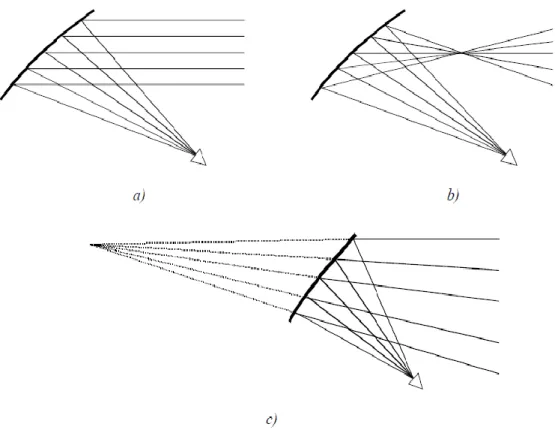+7

Viittaukset

LIITTYVÄT TIEDOSTOT

The electric field distribution of the reflected (the +z half-space) and transmitted (the −z half-space) waves normalized to the electric field of the incident wave: (a) for

Keywords: silicon edgeless detector, scanning electron microscope, TOTEM experiment, electric field distribution.. The main idea of this diploma work is to study electric

Stora Enso WSF. Stora Enso Wood Supply Finland. Environmental segmentation alternatives: a look at green consumer behaviour in the new millennium. Journal of Consumer

The insights developed in TRANS- included a different experience of a choreographic object which emerges in the light of choreographic practice rather than being pre-set, it

More specifically, with our systematic two-phase numerical evaluation, we demonstrated the benefits of DoA and ToA- DoA positioning technologies for improved communication

P redictive M obility -A ware C hannel C haracterization In the use cases discussed above, there are essentially two main data transfer paths: (i) an infrastructure link, where a

Figure 5.13: Electric potential, Electric field, logarithm of the gradient of the electric field square and DEP force images for 50 V pp for orientation 4 in model 3.1. The required

5.) Rapid changes of the ionospheric and magnetospheric electric currents cause variation in the geomagnetic field which according to Faraday’s law of induction induce an electric

- Gewinnung von Milchsäure und Protein aus Bioabfall Gewinnung von Protein. Schwarze Soldatenfliege